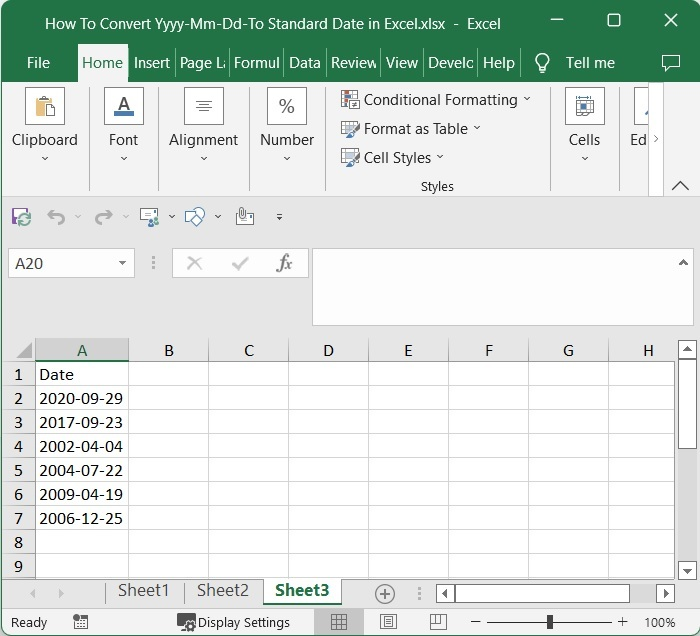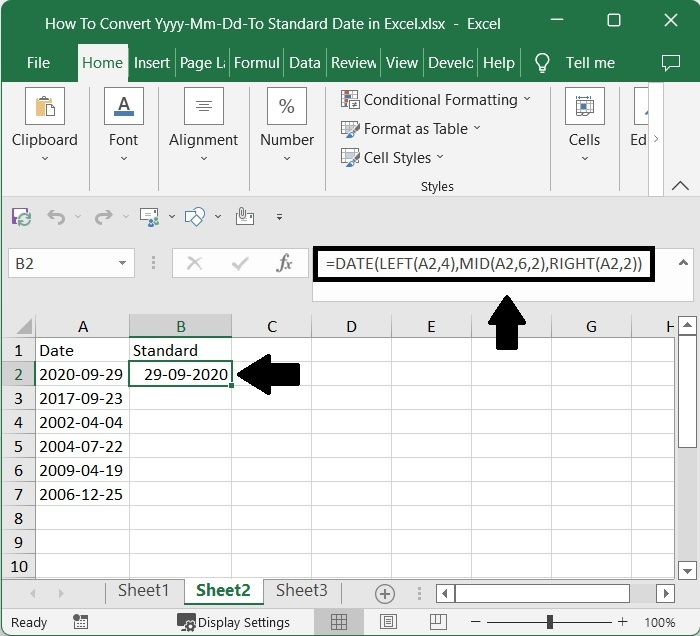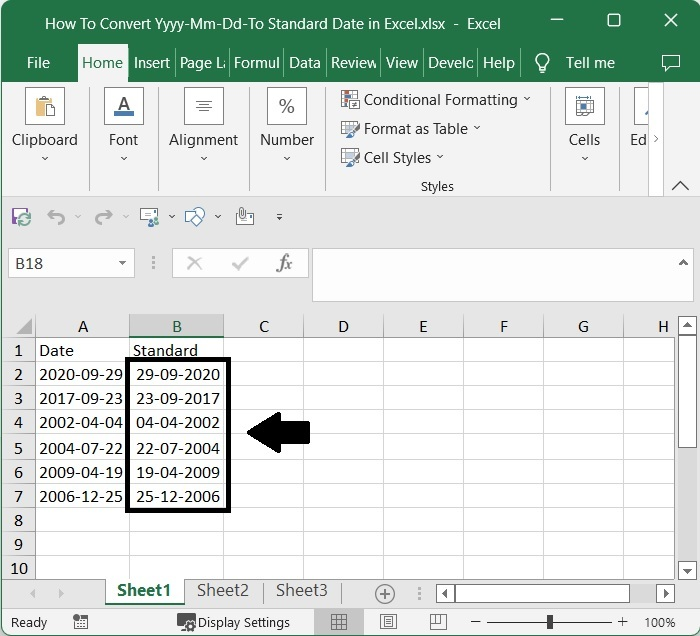# How to Convert YYYY-MM-DD to Standard Date in Excel?

In Excel, we always try to show the dates in the format of dd-mm-yyyy, which is the standard format when we use the dates in Excel. However, when the date is given in a different format, some users may feel uneasy because they may have only used the standard format for a long time.

Generally, in Excel, when we enter any format of date in the sheet, it will be directly converted to the standard format. If we try to convert any other format to standard format manually, then it can be a time-consuming and inaccurate process as we need to change every date manually.

Read this tutorial to learn how you can convert the yyyy-mm-dd format to a standard format in Excel. We can complete the task using the formulas supported in Excel.

## Converting YYYY-MM-DD to Standard Date in Excel

Here, we will first use the formula to get any one of the results, then use the auto-fill handle to get all the results. Let's go over a simple procedure for converting yyyy-mm-dd to standard format using Excel formulas. We can complete the task using the Date function in Excel.

### Step 1

Let us consider an excel sheet where the data is a date in the yyyy-mm-dd format, similar to the below image.Now, in cell B2, click on an empty cell and enter the formula as follows −

=DATE(LEFT(A2,4), MID(A2,6,2), RIGHT(A2,2)) and press enter to get our first result, which looks like the image below. In the formula, A2 is the address of our value on the sheet.

Empty cell > Formula > EnterIn the formula A2 is the address of our value on the sheet. in stands for inches and ft stands for feet. We can convert the values in to any metrics just by changing the names in formula/

### Step 2

Then, to get all the results, drag down from the first result using the auto-fill handle, and our final result will be similar to the below image.Note − We can also use the formula as follows